# From Geometric Mean to KL Divergence to Noisy Labels

If you have ever participated in a machine learning competition (like those held by Kaggle), you may be familiar with the geometric mean as a simple method that combines the output of multiple models. In this post, I will review the geometric mean and its relation to the Kullback–Leibler (KL) divergence. Based on this relation, we will see why the geometric mean might be a good approach for model combination. Then, I will show how you can build upon this relation to develop a simple framework for cleaning noisy labels or training machine learning models from noisy labeled data.

#### Geometric Mean for Classifier Fusion

Imagine you would like to build an image classification model to categorize cats, dogs, and people in your personal images. One strategy to achieve the best classification accuracy is to fuse the output of multiple models trained on your dataset. Let’s say you have trained three convolutional neural networks (CNNs) to classify images into these three categories. The CNNs may predict the following probability values over the categories for an image: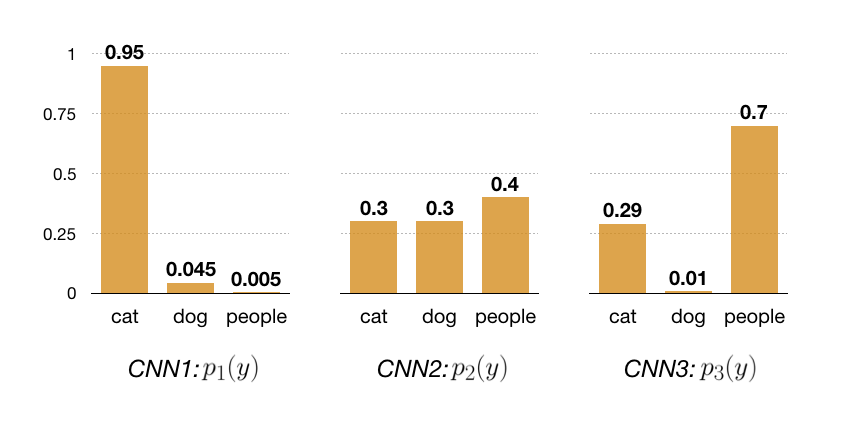But, how can we aggregate the output of the CNNs to produce a single prediction for the image? For this example, you may use majority voting which will assign the image to the people category, as 2 out of 3 CNNs predicted this category. However, the majority vote does not consider the confidence of each classifier and it ignores that CNN2 is slightly leaning towards people while CNN1 is highly confident against this category.

In practice, a better approach is to use the geometric mean of the predicted values to construct a distribution over the categories. We will represent this using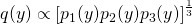whereindicates that the distribution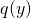is proportional to the geometric mean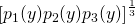. Let’s see how we can calculate this: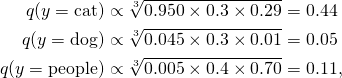where after normalization we have: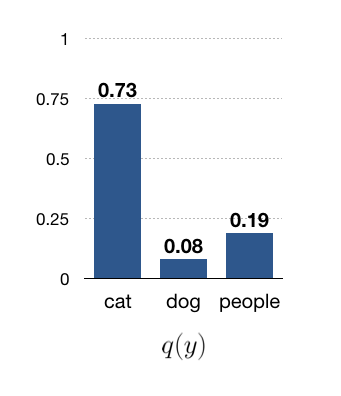As you can see, the geometric mean chooses the cat category instead of people, as CNN1 is highly confident on this cateory while other CNNs have given a moderate probablity to this category.

We can replace the geometric mean with the weighted geometric mean represented by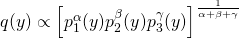where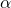,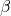, and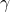are positive scalars controling the impract of each distribution on the final distribution. This is useful for example when you know CNN1 is more accurate than CNN2 and CNN3. By settinggreater thanand, you can force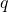to be closer to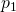.

#### Geometric Mean and KL Divergence

The KL divergence is a measure commonly used to characterize how a probability distributions differs from another distribution. Instead of aggregating the classification predictions using the geometric mean, we could use the KL divergence to find a distributionthat is closest to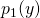,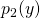and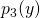. If suchis found, we can use it for making the final prediction as it is close to all the distributions,and. This is to say we solve:

(1)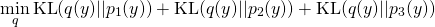Using the definition of the KL divergence, we can expand the above objective to:

(2)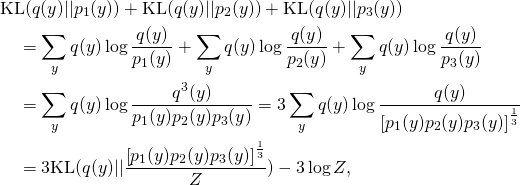where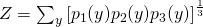is the normalization constant that makes the geometric mean distribution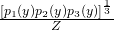a valid distribution. Above, we have converted the KL summation in (1) to a single KL in (2). It is easy to see that the KL in (2) is minimized ifis proportional to. This is an interesting result. The geometric mean of,andis a distribution close to all three based on the KL objective in (1). This may explain why the geometric mean is a good approach to classifier fusion. It simply finds the closest distribution to all the predictions.

Similarly, we can show that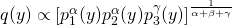, the weighted geometric mean, is the solution to the weighted KL objective: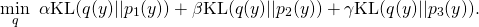#### From KL Divergence to Noisy Labels

Let’s now consider the problem of training an image classification model from noisy labeled images. Again, the categories that we are considering are cat, dog, and people. But, our training annotations are noisy, and we would like to develop a simple approach to clean the training annotations and use them for training. Here, we will see how we can use the geometric mean and equivalently the KL divergence to develop such an approach.

Let’s assume we can estimate transition probablities from noisy to clean labels. This can be denoted by: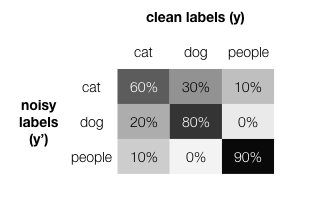This matrix represents the conditional distribution on a clean label (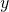) given a noisy label (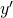). For example, the top-left entry indicates that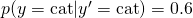, i.e., if an image is labeled as cat, with 60% probablity the true label is also cat.

The transition probabilities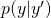, provided by the matrix, form a distribution over true labels for each noisy labeled instance. Instead of using the noisy annotations, we can use the probabilistic labels provided byto train the image classification model. For example, if an image is annotated by cat, we can assume that the true label is cat with probability 0.6, dog with probability 0.3, and people with probability 0.1. Although this approach provides robustness to label noise to some degrees, it does so by only considering the dependency between noisy and clean labels, independent of the image content, i.e., it does not infer the true labels specifically for each image. This type of noise correction is known as the class conditional noise model (see this paper for a theoretical discussion).

When we are training an image classification model, the model itself may be able to successfully predict the true labels (or at least a reasonable distribution over true labels) given a training image. In this case, we can use the model to infer a distribution over true labels. Let’s denote the classification model that is being trained by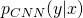which predicts a distribution over true labels given the image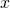. We can use the KL divergence below to find the closest distribution to bothand:

(3)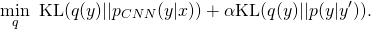where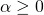is a scalar. At early training stages,cannot predict the true labels correctly. We can setto a large value such thatis only close to. As the classification model is trained, we can decreaseto letbe close to both distributions.

There are two advantages to the KL minimization in (3): i) The global solution can be obtained forusing the geometric mean (as shown above). Thus,can be inferred efficiently at each training iteration given the current modeland. ii) Aspredicts the labels given the image content,can be considered as an image-dependent noise correction model. In fact,can be used to infer the true labels for each noisy-labeled instance.

The idea of combining an auxiliary source of information (such as) and the underlying classification modelwas first introduced in this paper where I showed that the KL minimization in (3) is the natural result of a regualarized EM algorithm.

The original paper only considered binary class labels. Here, we extended this model to continuous labels (i.e., object location) and we showed that robust object detection models can be developed using this simple idea. Also, we showed that more sophisticated auxiliary sources of information can be formed for the object detection problem using image classification models.

Last but not least, the geometric mean expression used for inferringcan be considered as a linear function applied to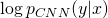and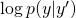. Here, we show that better inference models can be designed by training a CNN to representinstead of a fixed linear function. We show that this model is effective in the image segmentation problem.

Use the subscription form below if you’d like to stay tuned with the future posts:

### 2 thoughts on “From Geometric Mean to KL Divergence to Noisy Labels”

1.While you expanded the objective using KL definition , I was trying to reformulate and redo it from a scratch … but i stumbled between step 2 and step 3 when you included the Z term and i could not find what you found .It seems that there is a missing term q(y) : …. – q(y) x 3 Log Z
I did not get how did you get rid of q(y) because it seems to be a common multiplier before transforming the division inside the log into a substraction

1.You are on the right track. The reason I can get rid of q(y) in “\sum_y q(y) 3\log Z” is that “\log Z” is a constant that depends on p_1, p_2, and p_3. It’s not a function of y. So, \log Z comes out of the summation and we know \sum_y q(y) = 1 for any distribution.